HarDNet : A Low Memory Traffic network 를 읽고 개인적으로 정리한 글입니다.

# HarDNet : A Low Memory Traffic network (ICCV 2019)

## Key Idea

• 기존의 metrics들에서의 inference time 측정은 부정확하다.
• 새로운 metric => memory traffic for accessing intermediate feature maps 측정
• inference latency 측정에 유용할 것, especially in such tasks as real-time object detection and semantic segmentation of high-resolution video.
• CIO : approximation of DRAM traffic이 될 수 있다.
• computation, energy efficiency를 위해서는 fewer MACs, less DRAM이 좋은 것임 -> 연구 방향
• 각각의 레이어의 MoC에 soft constraint를 적용했음.
• low CIO network model with a reasonable increase of MACs를 위해
• 방법 -> avoid to employ a layer with a very low MoC such as a Conv1x1 layer that has a very large input/output channel ratio.
• input/output channel ratio가 크면 low MoC를 가진다는 사실을 알 수 있음.
• Densely Connected Networks에 영감을 받아 모델 빌딩함.
1. DenseNet의 다수의 layer connections들을 줄였음. => concatenation cost를 줄이기 위해
2. balance the input/output channel ratio by increasing the channel width of a layer according to its connections.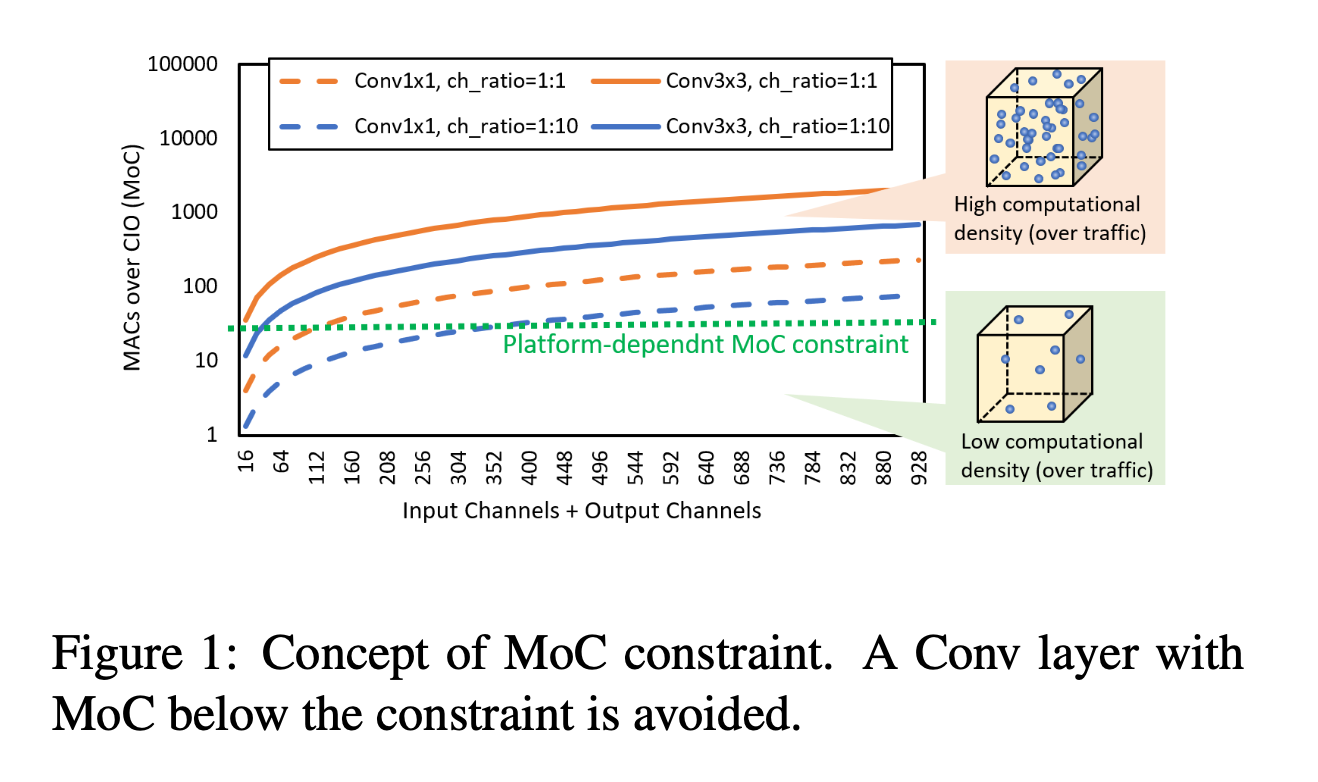• DRAM traffic

## Basic Concepts

• MAC : number of multiply-accumulate operations or floating point operations
• DRAM : Dynamic Random-Access Memory
• read/write model param. and feature maps
• CIO : Convolutional input/output
• 모든 conv layer에 대해 IN(C,W,H) X OUT(C,W,H) sum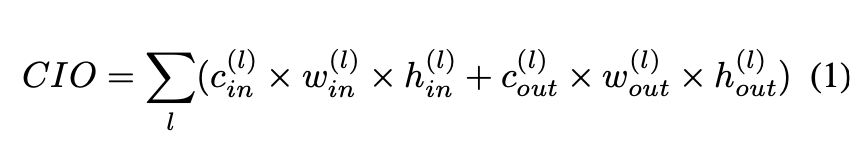• MoC : MACs over CIO of a layer = MACs/CIO
• TREND : exploiting shortcuts
• Highway networks, Residual Networks : add shortcuts to sum up a layer with multiple preceeding layers.
• DenseNet : concatenates all preceeding layers as a shortcut achieving more efficent deep supervision.
• 그러나 shortcuts는 large memory usage, heavy DRAM traffic을 유발할 수 있다.
• Using shortcuts elongates the lifetime of a tensor, which may result in frequent data exchanges betwwen DRAM and cache.

• DenseNet의 sparsified version : LogDenseNet, SparseNet
• Sparse
• The pros? If you have a lot of zeros, you don’t have to compute some multiplications, and you don’t have to store them. So you may gain on size and speed, for training and inference (more on this today).
• The cons? Of course, having all these zeros will probably have an impact on network accuracy/performance.
• increase the growth rate(output channel width) to recover the accuracy dropping from the connection pruning, and the increase of growth rate can compromise the CIO reduction
• 즉 increase of growth rate는 좋게 작용된다.

## Harmonic DenseNet

### Sparsification and weighting

• let layer k connect to layer k-2^n if 2^n divides k, where n is a non-negative integer and k-2^n >= 0
 12345678910111213141516171819 class HarDBlock(nn.Module): def get_link(self, layer, base_ch, growth_rate, grmul): if layer == 0: return base_ch, 0, [] out_channels = growth_rate link = [] for i in range(10): dv = 2 ** i if layer % dv == 0: k = layer - dv link.append(k) if i > 0: out_channels *= grmul out_channels = int(int(out_channels + 1) / 2) * 2 in_channels = 0 for i in link: ch,_,_ = self.get_link(i, base_ch, growth_rate, grmul) in_channels += ch return out_channels, in_channels, link 
• 2^n 개의 layer들이 이런 식으로 processed되면 layer [1 : 2^n -1]는 메모리에서 flush된다.
• 어떻게 flush 된다는 건지 잘 이해가 되지 않음.
• Power-of-two-th harmonic waves가 만들어짐. 그래서 Harmonic 이다.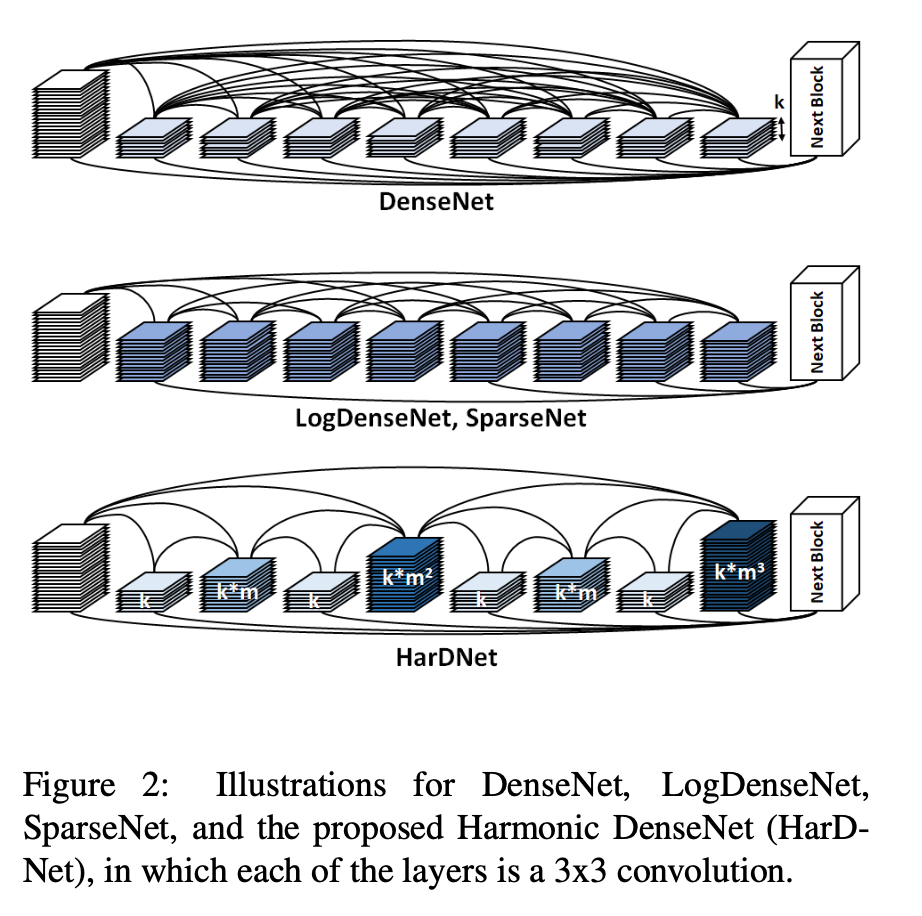• 이 방식은 concatenation cost를 눈에 띄게 감소시킨다.

• layers with an index divided by a larger power of two are more influential than those that divided by a smaller power of two.

• 많이 connection되니까 당연히 influential 하다.
• In this model, they amplify these key layers by increasing their channels, which can balance the channel ratio between the input and output of a layer to avoid a low MoC.
• 이런 key layer들을 amplify 했음(channel 수를 늘리면서)
• layer l has an initial growth rate k, we let its channel number to be k * m^n , where n is the max number satisfying that l is divided by 2^n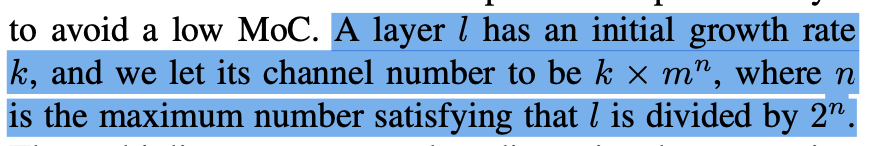• m 은 low-dimensional compression factor 역할을 한다.
• m 을 2보다 작게하면 input channel을 output channel보다 작게 할 수 있다.
• Empirically, settin m between 1.6 and 1.9

### Transition and Bottleneck Layers

• HDB(Harmonic Dense Block) : the proposed connection pattern forms a group of layers
• is followed by a Conv1x1 layer as a transition
• HDB의 depth는 2의 제곱수로 설정
• HDB의 마지막 레이어가 가장 큰 채널수를 가지도록 하기 위해서
• DenseNet -> gradient할 때 모든 레이어를 다 pass함
• 논문의 HBD with depth L -> pass through at most log L layers
• degradation을 완화시키기위해, depth-L HDB를 layer L과 all its preceeding odd numbered layers 를 concatenation시킨다.
• 2~L-2의 all even layer들의 아웃풋은 HDB가 한번 끝날때마다 버려진다.
• Bottleneck layer
• DenseNet에서는 param. efficiency를 위해 매 Conv3x3 layer전에 bottleneck을 두었다.
• 하지만 HarDnet에서는 위에서 이미 channel ratio(매 레이어마다 input&output 사이의)의 균형을 잡았으므로 bottleneck layer는 쓸모없어진다.
• 그래서 HBD에서는 Bottleneck layer없이 Conv3x3 for all layers
• Transition layer
•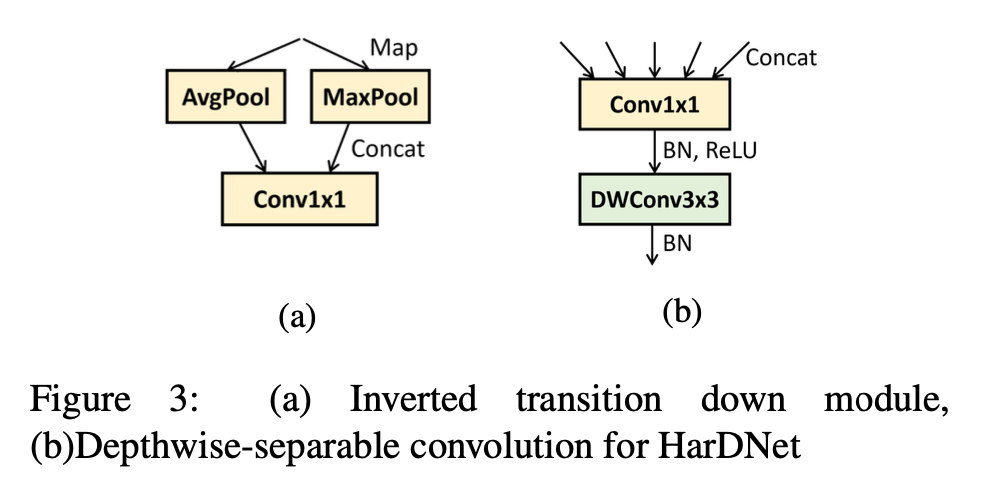• inverted trainsition module
• maps input tensor to an additional max pooling function along with the original average pooling, followed by concatenation and Conv1x1.
• 50% of CIO를 감소시킴

## Experiments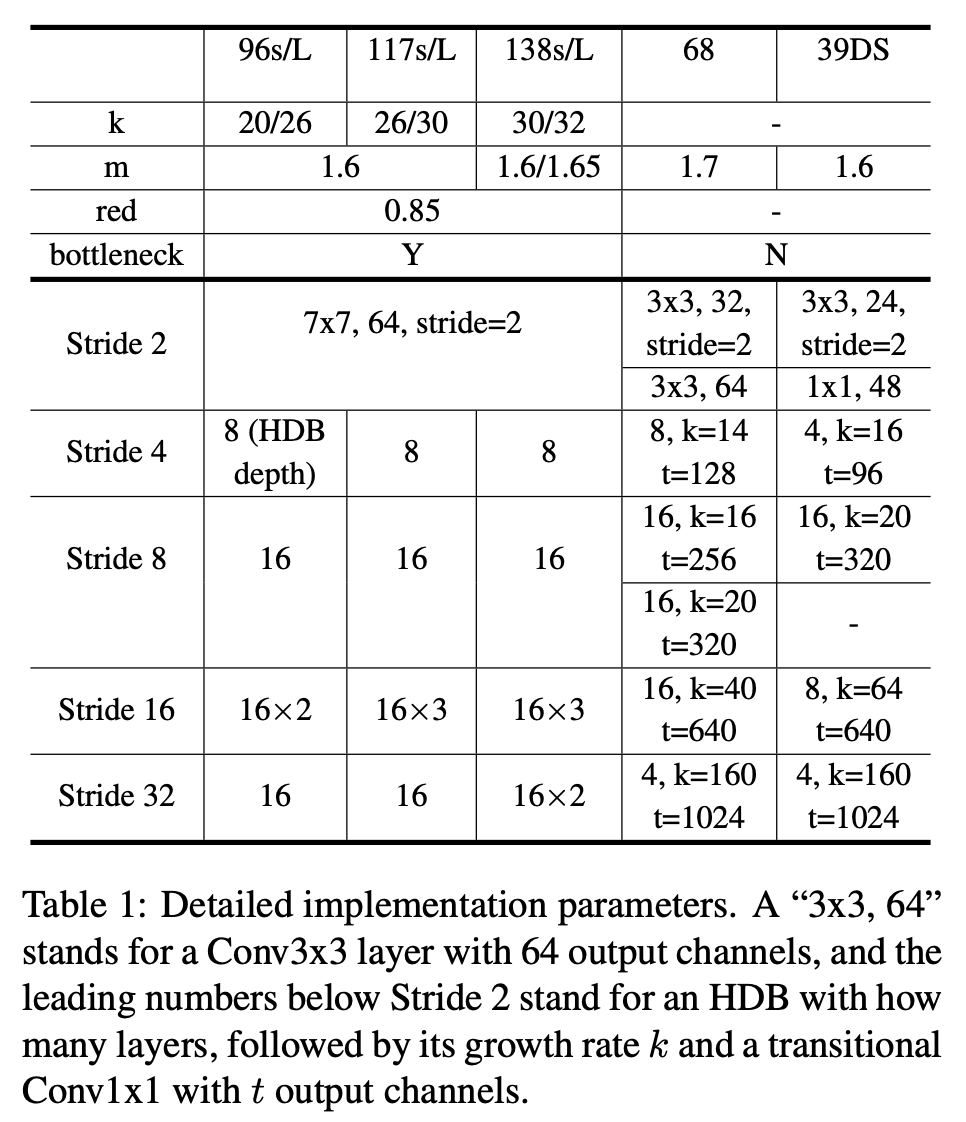• CamVid Dataset

• replace all the blocks in a FC-DenseNet with HDBs
• the architecture of FC-DenseNet with an encoder-decoder structure and block level shortcuts to create models for sematic segmentation.

We propose FC-HarDNet84 as specified in Table 3 for comparing with FC-DenseNet103. The new network achieves CIO reduction by 41% and GPU inference time reduction by 35%. A smaller version, FC-HarDNet68, also outperforms FC-DenseNet56 by a 65% less CIO and 52% less GPU inference time.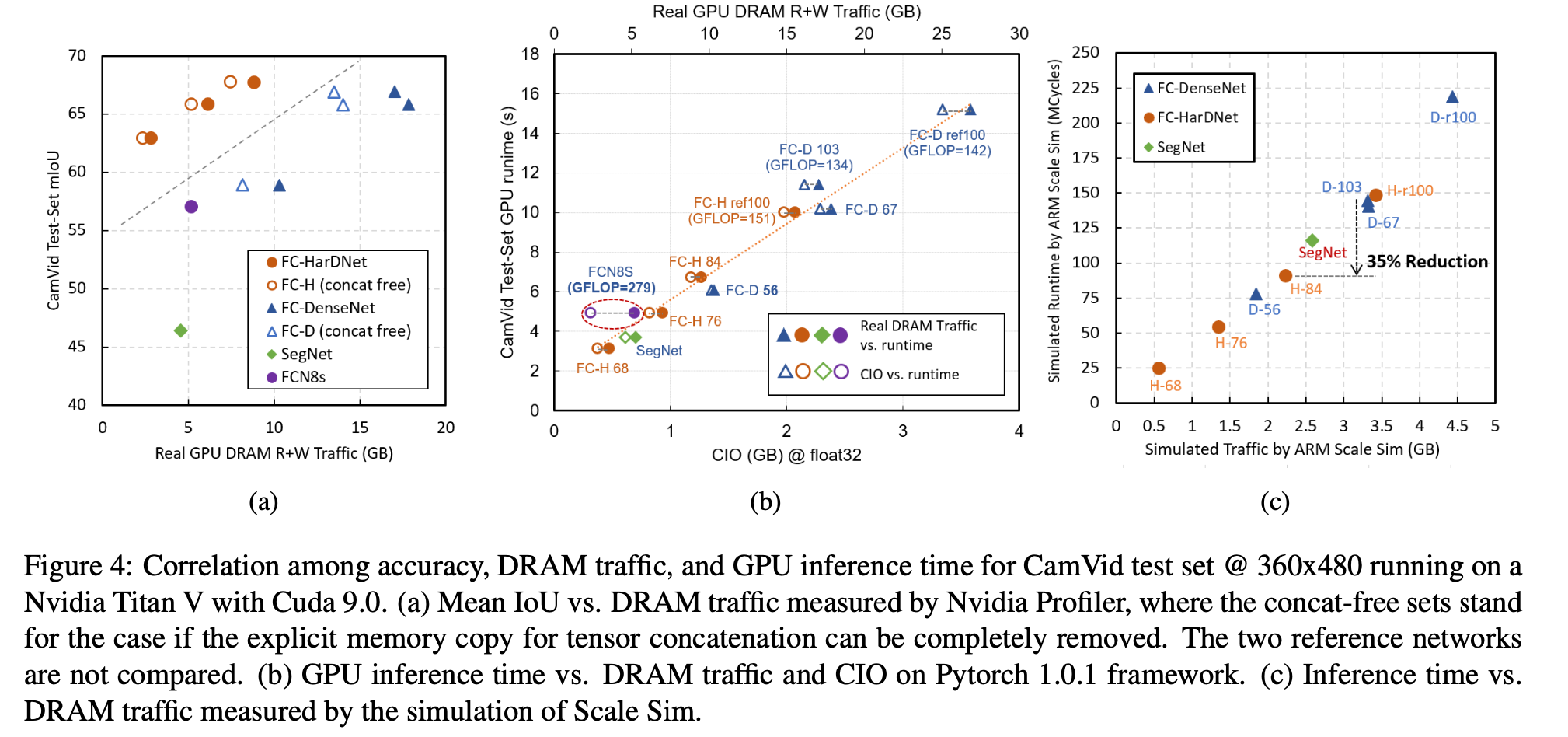• ImageNet Datasets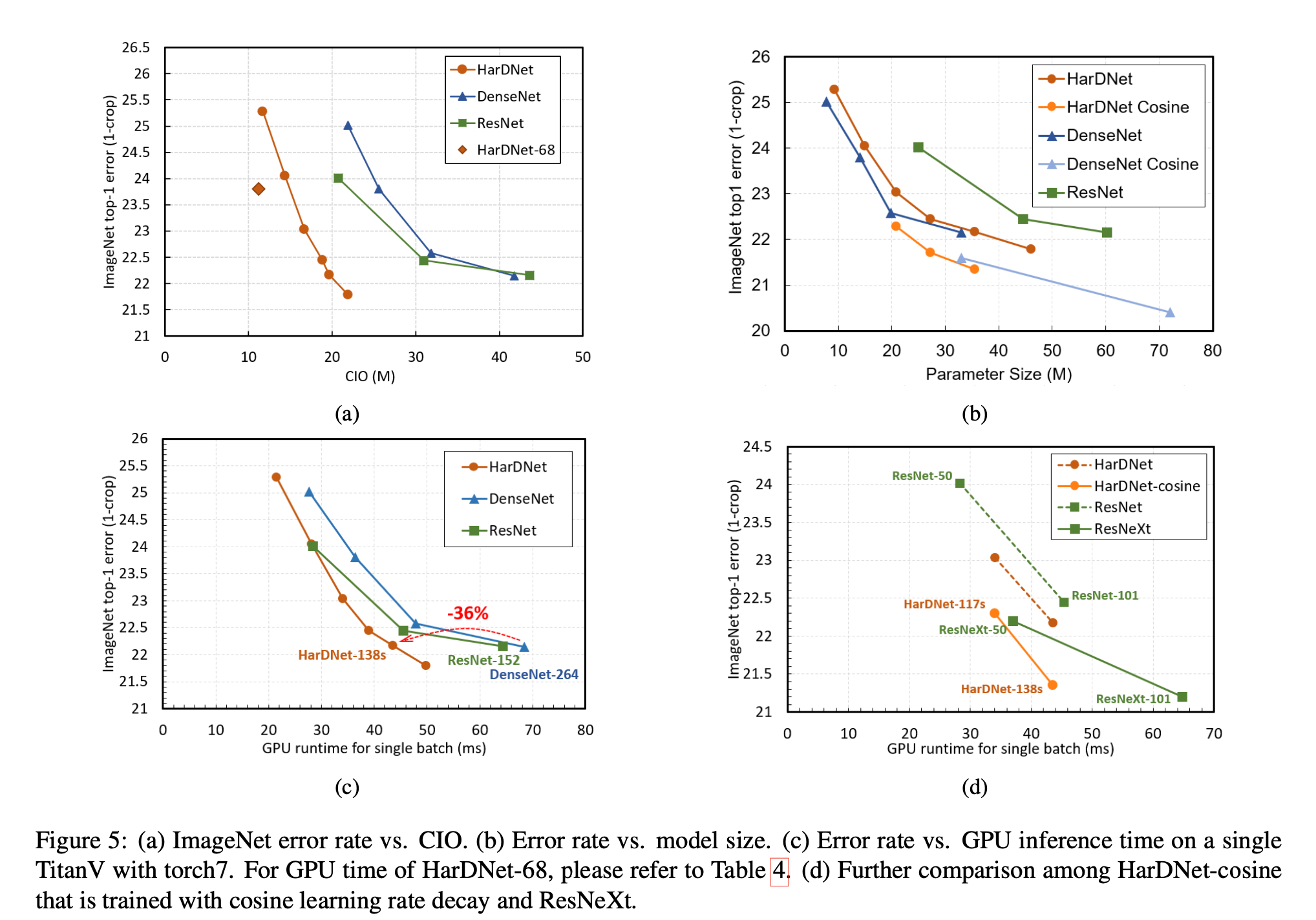• Object Detection
• HarDNet-68 as a backbone model for a Single Shot Detector (SSD) and train it with PASCAL VOC 2007 and MS COCO datasets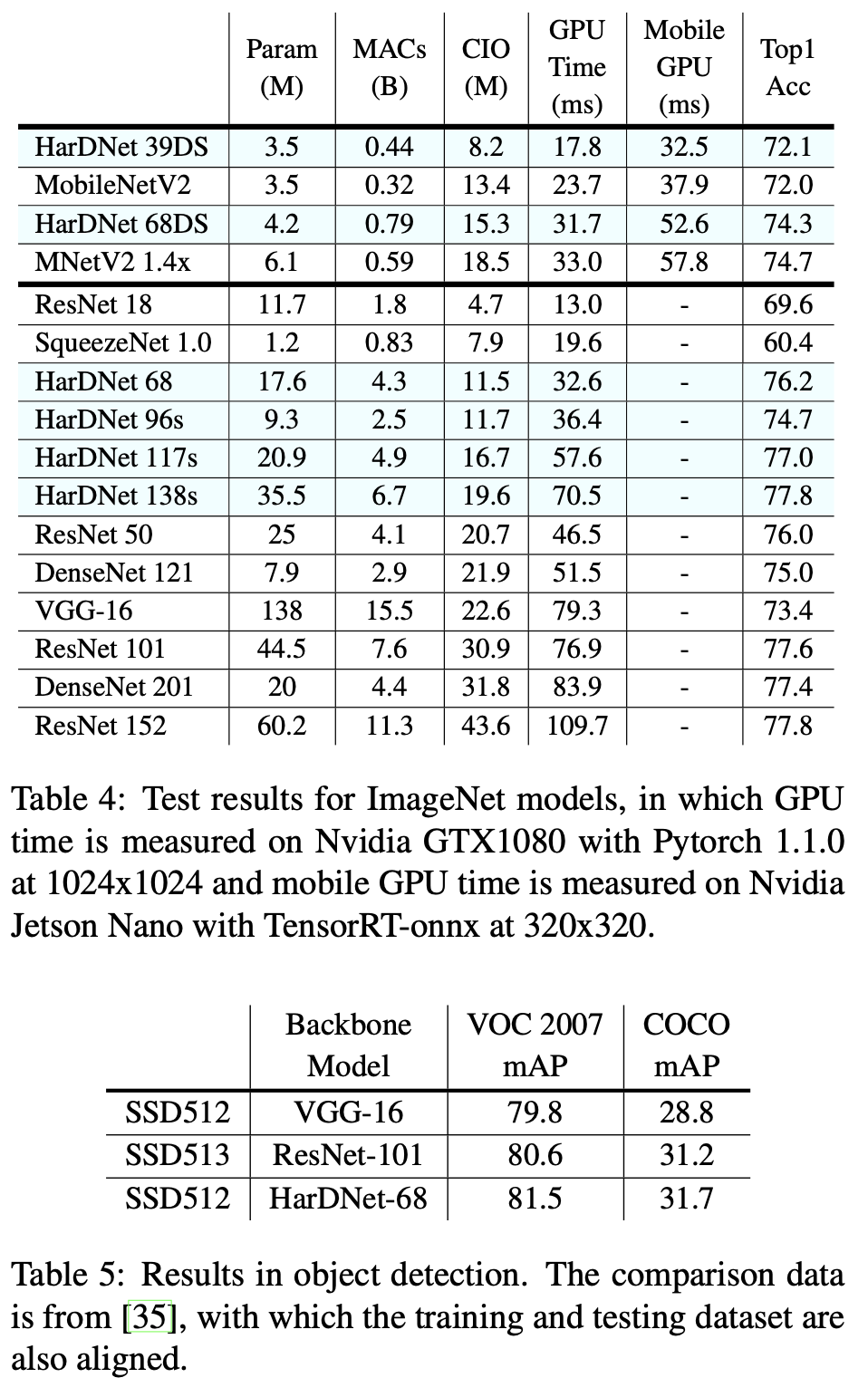## Discussion

There is an assumption with the CIO, which is a CNN model that is processed layer by layer without a fusion. In contrast, fused-layer computation for multiple convolutional layers has been proposed.

• CIO still failed to predict the actual inference time such as comparing two network models with significantly differnent architectures

In some of the layers CIO may dominate, but for the other layers, MACs can still be the key factor if its computational density is relatively higher. To precisely predict the inference latency of a network, we need to breakdown to each of the layers and investigate its MoC to predict the inference latency of the layer.

• 어쨌거나 DRAM traffic의 중요성을 강조하고 싶어함.
• traffic reduction을 위한 가장 좋은 방법은 MoC를 증가시키는 것
• which might be counter-intuitive to the widely-accepted knowledge of that using more Conv1x1 achieves a higher efficiency.